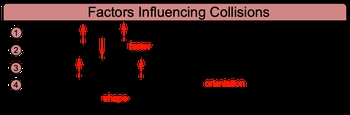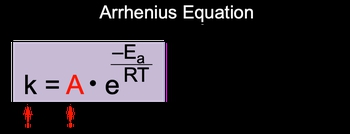## General Chemistry

Learn the toughest concepts covered in Chemistry with step-by-step video tutorials and practice problems by world-class tutors

15. Chemical Kinetics

# Collision Theory

According to Collision Theory:chemical reaction is successful when 2 energetic reactants successfully collide.

Understanding Collision Theory
1
concept

## Collision Theory Concept 12m
Play a video:
according to collision theory, a chemical reaction is successful when two energetic reactant successfully collide and when we talk about successfully collide, we're gonna say that for successful collisions, reactive molecules must collide with enough energy so they have to hit each other hard enough and with proper orientation so they have to hit each other in the right spots for them to stick together. Now, what are the factors that are influencing our collisions? Well, the first factor is temperature, we're gonna say here, if we can increase the temperature of our reaction, then this can help increase the number of energetic collisions. More energetic collisions. More success in possibly connecting together between reacting molecules. Now activation energy, if we can ensure that our chemical reaction has a low activation energy, then this will also increase our rate of reaction. Next concentration of reactivates. If you can increase the amount of reacting concentrations, that means they're gonna be more reacting molecules floating around, which will increase their frequencies of collision, increasing the frequencies of collisions. Mean that they bump into each other more often and that increases the possibility of some of them sticking together. Now four, we're going to talk about orientation. This is pretty self explanatory here we say, molecules must collide with proper orientation and if they do so, that'll equal a successful collision. Now here, proper orientation is partially determined by the shape of molecules. So for them to connect in the right spots, the molecules themselves have to have ideal shapes with one another. Now the rate of reaction is influenced by molecular collisions here, we're going to say that if we can increase the number of overall collisions, then this can result in a faster rate of reaction. So now keep all of these factors in mind when we're talking about successful collisions, which in turn will lead to a faster rate of reaction.

A chemical reaction is successful if reactant molecules can combine together to form a new product.2
example

## Collision Theory Example 11m
Play a video:
in this example question it says for chemical reaction to occur, all the following must happen. Except Alright, so here a large number of collisions must occur. Chemical bonds in reactions must break, reactive molecules must collide with correct orientation, reactive molecules must collide with enough energy or none of the above. All right, So let's make sure we take out the ones that we know have to happen. So here reactive molecules must collide with correct orientation. This is one of the foundations of collision theory we talked about. They have to basically connect in the right spots. So here this has to happen. What else must happen? Reacting molecules must collide with sufficient energy, enough energy. Now, here we have A B and E. So for B we say chemical bonds in reactant must break. While the whole point of these chemical reactions is to change reactant into products and reactant can only change into products if their bonds break, so be has to happen. The answer here is a yes, collisions need to occur for um them to stick together, but it's not necessary that it has to be a large enough number of collisions. Some reactions can happen with just a few collisions because they're highly favorable. So here, it would be the best answer. We need collisions. We don't always necessarily need a large number of collisions. Again, some reactions only need a few and they automatically stick together. Right, so here the final answer would be option a
3
concept

## Collision Theory Concept 22m
Play a video:
Now the Iranians equation illustrates how the rate of a reaction is affected by different variables. Now we're going to say here that a faster reaction rate would have a high K value or rate constant K. It would have a higher a variable, it would have a lower activation energy and it would have a higher temperature. Now here we're gonna incorporate these variables into our Arrhenius equation itself. In the Iranians equation we say that K. Which represents your rate constant equals A. Which represents your frequency factor times the inverse of the natural log to be negative E. A over R. T. Now here E. A. Is our activation energy are here represents our gas constant which is 8.314 jewels over moles times K, which is kelvin. And then here T equals temperature in kelvin with this radius equation rate Iranians equation, we can say here that the higher the rate constant K than the higher the rate of reaction or the faster the rate of reaction. Now with our frequency factor A, we can say that it is split into two other variables. And those variables are the orientation factor which uses the variable P. And the collision frequency, which is using the variable Z. Yeah. Now we can say here that our orientation factor P. It's just a number that represents the fractions of collisions with correct orientation here, we can say that the larger the reacting molecules or reactant than the lower the orientation factor and the lower the orientation factor, the less successful your collision will be with the collision frequency easy, that's just the frequency of molecular collisions that occur. So the higher your Z value, the more molecular collisions that can occur, the more likely some of them will be successful. So just keep in mind these basic ideas and principles when it comes to the Arrhenius equation, it's really just looking at how all the factors together can result in either a successful or unsuccessful reaction.

Frequency Factor is made up of 2 variables:

1. Orientation Factor (p)

2. Collision Frequency (z)4
example

## Collision Theory Example 21m
Play a video: